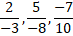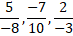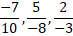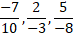# Quiz Discussion

The price of commodity X increases by 40 paise every year, while the price of commodity Y increases by 15 paise every year. If in 2001, the price of commodity X was Rs. 4.20 and that of Y was Rs. 6.30, in which year commodity X will cost 40 paise more than the commodity Y ?

Course Name: Quantitative Aptitude

• 1] 2010
• 2] 2011
• 3] 2012
• 4] 2013
##### Solution
No Solution Present Yet

#### Top 5 Similar Quiz - Based On AI&ML

Quiz Recommendation System API Link - https://fresherbell-quiz-api.herokuapp.com/fresherbell_quiz_api

# Quiz
1
Discuss

0.04 x 0.0162 is equal to:

• 1] 6.48 x 10-3
• 2] 6.48 x 10-4
• 3] 6.48 x 10-5
• 4] 6.48 x 10-6
##### Solution
2
Discuss

The value of $$\left( {\frac{{0.943 \times 0.943 - 0.943 \times 0.057 + 0.057 \times 0.057}}{{0.943 \times 0.943 \times 0.943 + 0.057 \times 0.057 \times 0.057}}} \right)$$ is :

• 1] 0.32
• 2] 0.886
• 3] 1.1286
• 4] None of these
##### Solution
3
Discuss

47.7 × 12.4 × 8.6 = ?

• 1] 5086.728
• 2] 5218.668
• 3] 5708.428
• 4] 6180.656
• 5] None of these
##### Solution
4
Discuss

Which of the following fractions lies between 2/3 and 3/5 = ?

• 1]

2/5

• 2]

1/3

• 3]

1/15

• 4]

31/50

##### Solution
5
Discuss

$$\frac{{4.2 \times 4.2 - 1.9 \times 1.9}}{{2.3 \times 6.1}}$$    is equal to:

• 1] 0.5
• 2] 1
• 3] 20
• 4] 22
##### Solution
6
Discuss

Solve $$\frac{294 ÷ 14 × 5 + 11}{?}$$    = 82 ÷ 5 + 1.7

• 1] 8
• 2] 6
• 3] 12
• 4] 5
• 5] 10
##### Solution
7
Discuss

The value of $$\frac{{489.1375 \times 0.0483 \times 1.956}}{{0.0873 \times 92.581 \times 99.749}}$$     is closest to:

• 1] 0.006
• 2] 0.06
• 3] 0.6
• 4] 6
##### Solution
8
Discuss

The rational numbers lying between 1/3 and 3/4 are :

• 1]

$$\frac{{117}}{{300}},\frac{{287}}{{400}}$$

• 2]

$$\frac{{95}}{{300}},\frac{{301}}{{400}}$$

• 3]

$$\frac{{99}}{{300}},\frac{{301}}{{400}}$$

• 4]

$$\frac{{97}}{{300}},\frac{{299}}{{500}}$$

##### Solution
9
Discuss

Solve : $$7\frac{1}{2} - \left[ {2\frac{1}{4} ÷ \left\{ {1\frac{1}{4} - \frac{1}{2}\left( {1\frac{1}{2} - \frac{1}{3} - \frac{1}{6}} \right)} \right\}} \right]$$       = ?

• 1]

2/9

• 2]

$$4\frac{1}{2}$$

• 3]

$$9\frac{1}{2}$$

• 4]

$$1\frac{77}{228}$$

##### Solution
10
Discuss

The arrangement of rational numbers, -7/10, 5/-8, 2/-3  in ascending order is :

• 1]• 2]• 3]• 4]# Quiz